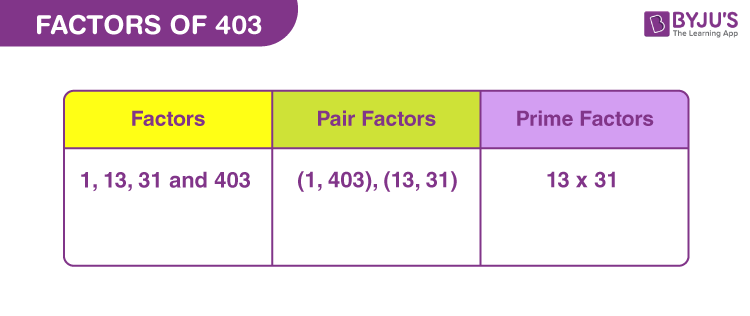# Factors of 403

Factors of 403, in Maths, are the natural numbers that can divide the original number, in a uniform manner. The factorisation of any number, results in the product form of factors. 403 is an odd number and is a composite number, also. A composite number, by definition, has more than two factors.

403 can also be expressed as the product of two factors that results in the original number. Such two factors are called pair factors. Also, the prime factorisation of a number is the product of prime numbers or prime factors. In this article, we will find all the factors of 403 along with their pair factors and prime factors.## How to Find the Factors of 403?

We can use the simple division method to find the factors of 403. In this method, we divide the actual number, by a set of natural numbers, such that the remainder is zero.

 403 ÷ 1 = 403 [Remainder = 0] 403 ÷ 13 = 31 [Remainder = 0] 403 ÷ 31 = 13 [Remainder = 0] 403 ÷ 403 = 1 [Remainder = 0]

Therefore, there are four factors of 403. They are 1, 13, 31 and 403.

## Pair Factors of 403

The factors in pairs, when multiplied together, result in the original number. Therefore, the pair factors of 403 are:

• 1 × 403 = 403
• 13 × 31 = 403

Or

• 403 × 1 = 403
• 31 × 13 = 403

Therefore, the pair factors of 403 are (1, 403) and (13, 31).

Now, if we consider negative pair factors , they will result in the actual number, after multiplication.

• -1 × -403 = 403
• -13 × -31 = 403

Therefore, the negative pair factors are (-1, -403) or (-13, -31).

## Prime Factorisation of 403

The prime factors of 403 are the prime numbers that can be determined by the prime factorisation method.

Step 1: Divide 403 by the prime number 13

403/13 = 31

Step 2: Divide 31 by itself

31/31 = 1

Therefore, the prime factorisation of 403 is 13 x 31 and the prime factors of 403 are 13 and 31.

 Prime factorisation of 403 = 13 x 31 Exponential form = 131 x 311

## Solved Examples

Q.1: What is the sum of factors of 403?

Solution: The factors of 403 are 1, 13, 31 and 403.

Sum = 1 + 13 + 31 + 403 = 448

Therefore, the sum of factors of 403 is 448.

Q.2: Is 403 divisible by 3, evenly?

Solution: If we divide 403 by 3, then we get:

403/3 = 134.333…

Since a fraction cannot be a factor of a natural number, therefore 403 is not divisible by 3.

Q.3: What is the GCF of 403, 434, and 465?

Solution: The factors of 403, 434 and 465, respectively, are:

403 → 1, 13, 31, 403

434 → 1, 2, 7, 14, 31, 62, 217, 434

465 → 1, 3, 5, 15, 31, 93, 155, 465

Therefore, the GCF of 403, 434 and 465 is 31.

Q.4: What are the common factors of 186 and 403?

Solution: The factors of 186 and 403, respectively, are:

186 → 1, 2, 3, 6, 31, 62, 93, 186

403 → 1, 13, 31, 403

Therefore, the common factors of 186 and 403 are 1 and 31.

Q.5: What is the LCM of 186 and 403?

Solution: The LCM or Least common multiple can be determined by listing the factors or prime factorisation method.

Prime factorisation of 186 = 2 x 3 x 31

Prime factorisation of 403 = 13 x 31

Therefore, LCM of 186 and 403 = 2 x 3 x 13 x 31 = 2418

### Practice Questions

1. What is the average of factors of 403?
2. What is the GCF of 303 and 403?
3. How many factors does the number 403 have?
4. Is 7 a factor of 403?

## Frequently Asked Questions on Factors of 403

### What are the factors of 403?

The factors of 403 are 1, 13, 31 and 403.

### What is the LCM of 403 and 408?

We can use the prime factorisation method to find the LCM of 403 and 408.

403 → 13 x 31

408 → 2 x 2 x 2 x 3 x 17

LCM = 2 x 2 x 2 x 3 x 13 x 17 x 31 = 164424

### Is 403 a prime number?

403 is not a prime number but a composite number.

### What are the prime factors of 403?

The prime factors of 403 are 13 and 31.

### What are the multiples of 403?

The multiples of 403 are 403, 806, 1209, 1612, 2015, 2418, 2821, 3224, 3627, 4030 and so on.